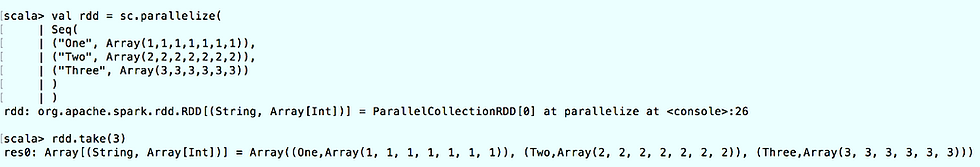top of page
BlogPageTop

# How to convert RDD to Dataframe?

Updated: Oct 25, 2019There are basically three methods by which we can convert a RDD into Dataframe. I am using spark shell to demonstrate these examples. Open spark-shell and import the libraries which are needed to run our code.

Scala> import org.apache.spark.sql.{Row, SparkSession}

Scala> import org.apache.spark.sql.types.{IntegerType, DoubleType, StringType, StructField, StructType}Now, create a sample RDD with parallelize method.

Scala> val rdd = sc.parallelize(

Seq(

("One", Array(1,1,1,1,1,1,1)),

("Two", Array(2,2,2,2,2,2,2)),

("Three", Array(3,3,3,3,3,3))

) )### Method 1

If you don't need header, you can directly create it with RDD as input parameter to createDataFrame method.

Scala> val df1 = spark.createDataFrame(rdd)### Method 2

Scala> val df2 = spark.createDataFrame(rdd).toDF("Label", "Values")### Method 3

If you need schema structure then you need RDD of [Row] type. Let's create a new rowsRDD for this scenario.

Scala> val rowsRDD = sc.parallelize(

Seq(

Row("One",1,1.0),

Row("Two",2,2.0),

Row("Three",3,3.0),

Row("Four",4,4.0),

Row("Five",5,5.0)

)

)Now create the schema with the field names which you need.

Scala> val schema = new StructType().Now create the dataframe with rowsRDD & schema and show dataframe.

Scala> val df3 = spark.createDataFrame(rowsRDD, schema)Thank you folks! If you have any question please mention in comments section below.

### Next: Writing data files in Spark

1. Apache Spark and Scala Installation

2. Getting Familiar with Scala IDE

3. Spark data structure basics

4. Spark Shell

5. Reading data files in Spark

6. Writing data files in Spark

7. Spark streaming